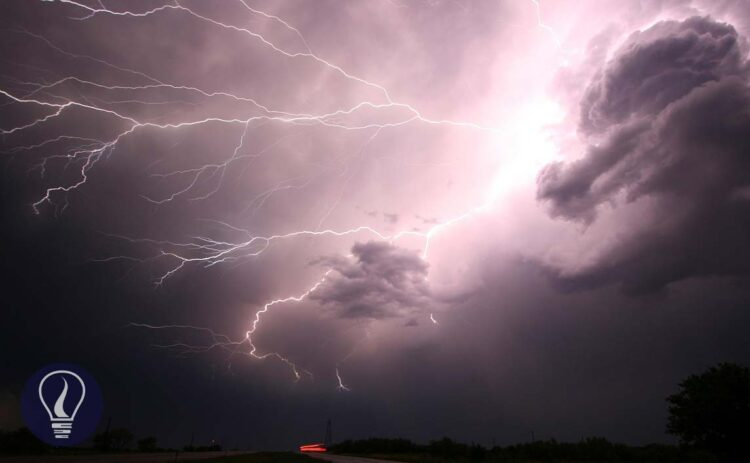More results...

Generic selectors
Exact matches only
Search in title
Search in content
Post Type Selectors

# Electric Field IntensityB.Sc (Hons, USJ) (Polymer Science and Technology, Chemistry, Physics)
Categories
Last Updated On : September 22, 2023
Published Date : September 21, 2023

An electric field is the space around a charged particle or an object, which is influenced by the charge of the charged particle or the object. Electric field intensity is the force acting on a charge when it is placed under an electric field.

When a charged particle with q charge is placed under an electric field if the force acting on the charged particle is F, mathematically the electric field intensity (E) is written as follows,

Where,

• E = Electric field intensity
• q = charge of the charged particle
• F = Force acting on the charged particle

Si unit of the electric field intensity is N C-1 (Newton per Coulomb) or Vm-1 (Volt per meter).

Example 01

When an electron is placed on an electric field, the force acting on the electron is 0.006 N. What is the electric field intensity where the electron is?

Solution

Example 02

What is the force that acts on a pointed charge with +2μC when it is placed on an electric field with the intensity of 1 Vm-1?

Solution

The force acting on the charged particle can be found using Coulomb's law. Therefore, we can rewrite the formula for E in terms of Coulomb's force.

When two charged particles, each having q charge are placed in an electric field with a displacement of r, the Coulomb's force would be,

E around one charged particle is,

Example 03

What is the electric intensity at a 2 cm distance from a proton, which is placed in a vacuum?

Solution

## Electric field intensity around a pointed charge

The electric field intensity (E) of any pointed charge that is placed in an electric field can be calculated using Coulomb's law. E at any point around a pointed charged particle is the electric force acting when a charge of +1 C is placed at the considered point. Mathematically,

If the pointed charge does not change, the electric field intensity is inversely proportional to the square of the displacement.

Example 04

The charge of a static pointed charge which is placed in a vacuum is +2C. Find the percentage decrease in E on moving from a distance of 1cm to a distance of 2cm from the pointed charge.

Solution

The E is inversely proportional to the square of the distance. The E at a 1 cm distance from the pointed charge,

The E at a 2 cm distance from the pointed charge,

Percentage decrease in E,## References and Attributes

### Figures:

The cover image was designed using an image by WikimediaImages from Pixabay